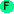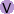# Variables

## Variablesdo [statement ...] Executes the statements in order. The do statement is useful when multiple statements need to be executed in a context that permits only a single statement, such as an 'if' clause. ```>(do (= x 2) (+ x 3)) 5 ```do1 expr [body ...] Saves the first expression and returns it after executing the body. ```>(let x 42 (do1 x (= x 50))) 42 ```def name params [body ...] Defines a function with the given name, taking the specified parameters. This is the standard way of creating a new function in Arc. The parameter list params can be an empty list (for a function that takes no arguments), a variable (which will hold an arbitrary number of parameters as a list), or a dotted list (with the last variable holding any left-over parameters as a list). ```>(do (def foo x (prn x)) (foo 1 2 3)) (1 2 3) (1 2 3) ``` ```>(do (def bar (a b c) (prn a "," b "," c)) (bar 1 2 3)) 1,2,3 1 ``` ```>(do (def baz (a . rest) (prn a "," rest)) (baz 1 2 3)) 1,(2 3) 1 ```defs [name params body] ... Performs multiple def operations. New in arc3. ```>(def foo x (prn x) bar (a b c) (prn a "." b "." c)) # ```defmemo name parms [body ...] Creates a memoized function.memo f Creates a memoized function from f.safeset var value Sets var to value. This is similar to =, except it prints a warning to stderr if var is already defined. ```>(do (safeset var 1) (safeset var 2)) 2 ```with ([var val ...]) [body ...] Creates a new variable binding and executes the body. The values are computed before any of the assignments are done (like Scheme's `let`, rather than `let*`). If the last variable doesn't have a value, it is assigned nil. ```>(with (a 1 b 2 c 3) (+ a b c)) 6 ```let var val [body ...] The let statement sets the variable var to the value within the scope of the body. Outside the let statement, any existing value of var is unaffected. Let is like `with` but with a single variable binding. ```>(let x 3 (+ x 1)) 4 ```withs ([var val ...]) [body ...] Creates a new variable binding and executes the body. The values are computed sequentially (like Scheme's `let*`, rather than `let`). If the last variable doesn't have a value, it is assigned nil. ```>(withs (a 1 b (+ a 1)) (+ a b)) 3 ```fn args [body ...] fn is used to create lambda functions. The args can be a variable (which will pick up all the arguments as a list), a list of variables, or a dotted list of variables (the last will pick up the remainder as a list ). ```>((fn (x y) (+ x y)) 1 2) 3 ``` ```>((fn all (len all)) 1 2 3) 3 ``` ```>((fn (arg1 arg2 . rest) rest) 1 2 3 4) (3 4) ```in x [choices ...] This predicate returns true if x is in the choices. Note that each choice is a separate argument. ```>(in 1 2 "b" 1 #\c) t ``` ```>(in 1 '(1 2 3)) nil ```copy x [key val] ... Copies x, updating entries corresponding to the args.. The input must be of type sym, cons, string, or table. For a sym, additional arguments are not permitted. For a list, the keys are numeric indices into the list, and the corresponding entries are replaced with the values. For a string, the keys are indices into the string and the values must be characters. For a table, the keys and values add or update entries in the table. Original object x is unmodified.sig Hash table containing function signatures. ```>(sig 'map) (f . seqs) ```defhook name [body ...] Creates a function (similar to def), except the function is registered in hooks*hook name [arg ...] Executes a function registered in hooks*hooks* (tests)